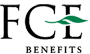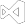Convert XLSX Spreadsheet File Types
``````using IronXL;
using System.IO;

// Import any XLSX, XLS, XLSM, XLTX, CSV and TSV

// Export the excel file as XLS, XLSX, XLSM, CSV, TSV, JSON, XML
workBook.SaveAs("sample.xls");
workBook.SaveAs("sample.xlsx");
workBook.SaveAs("sample.tsv");
workBook.SaveAsCsv("sample.csv");
workBook.SaveAsJson("sample.json");
workBook.SaveAsXml("sample.xml");

// Export the excel file as Html, Html string
workBook.ExportToHtml("sample.html");
string htmlString = workBook.ExportToHtmlString();

// Export the excel file as Binary, Byte array, Data set, Stream
byte[] binary = workBook.ToBinary();
byte[] byteArray = workBook.ToByteArray();
System.Data.DataSet dataSet = workBook.ToDataSet(); // Allow easy integration with DataGrids, SQL and EF
Stream stream = workBook.ToStream();``````
``````Imports IronXL
Imports System.IO

' Import any XLSX, XLS, XLSM, XLTX, CSV and TSV
Private workBook As WorkBook = WorkBook.Load("sample.xlsx")

' Export the excel file as XLS, XLSX, XLSM, CSV, TSV, JSON, XML
workBook.SaveAs("sample.xls")
workBook.SaveAs("sample.xlsx")
workBook.SaveAs("sample.tsv")
workBook.SaveAsCsv("sample.csv")
workBook.SaveAsJson("sample.json")
workBook.SaveAsXml("sample.xml")

' Export the excel file as Html, Html string
workBook.ExportToHtml("sample.html")
Dim htmlString As String = workBook.ExportToHtmlString()

' Export the excel file as Binary, Byte array, Data set, Stream
Dim binary() As Byte = workBook.ToBinary()
Dim byteArray() As Byte = workBook.ToByteArray()
Dim dataSet As System.Data.DataSet = workBook.ToDataSet() ' Allow easy integration with DataGrids, SQL and EF
Dim stream As Stream = workBook.ToStream()``````

The IronXL library enable a very convenience and safe way to convert from commonly used spreadsheet file format to the require format to be used in the next process of your .Net project. The available formats are shown below:

• XLS
• XLSX
• XLSM
• XLTX
• CSV
• TSV

### Export

• XLS, XLSX, XLSM, CSV and TSV
• JSON
• XML
• HTML
• In code data types:
• HTML string
• Binary
• Byte array
• Data set
• Memory stream

Exporting Excel into `System.Data.DataSet` and `System.Data.DataTable` objects allow easy interoperability or integration with DataGrids, SQL and EF.Read Excel Files without Interop
``````using IronXL;
using System;
using System.Linq;

// Supported for XLSX, XLS, XLSM, XLTX, CSV and TSV

// Select worksheet at index 0
WorkSheet workSheet = workBook.WorkSheets;

// Get any existing worksheet
WorkSheet firstSheet = workBook.DefaultWorkSheet;

// Select a cell and return the converted value
int cellValue = workSheet["A2"].IntValue;

// Read from ranges of cells elegantly.
foreach (var cell in workSheet["A2:A10"])
{
Console.WriteLine("Cell {0} has value '{1}'", cell.AddressString, cell.Text);
}

// Calculate aggregate values such as Min, Max and Sum
decimal sum = workSheet["A2:A10"].Sum();

// Linq compatible
decimal max = workSheet["A2:A10"].Max(c => c.DecimalValue);``````
``````Imports IronXL
Imports System
Imports System.Linq

' Supported for XLSX, XLS, XLSM, XLTX, CSV and TSV
Private workBook As WorkBook = WorkBook.Load("sample.xlsx")

' Select worksheet at index 0
Private workSheet As WorkSheet = workBook.WorkSheets(0)

' Get any existing worksheet
Private firstSheet As WorkSheet = workBook.DefaultWorkSheet

' Select a cell and return the converted value
Private cellValue As Integer = workSheet("A2").IntValue

' Read from ranges of cells elegantly.
For Each cell In workSheet("A2:A10")
Console.WriteLine("Cell {0} has value '{1}'", cell.AddressString, cell.Text)
Next cell

' Calculate aggregate values such as Min, Max and Sum
Dim sum As Decimal = workSheet("A2:A10").Sum()

' Linq compatible
Dim max As Decimal = workSheet("A2:A10").Max(Function(c) c.DecimalValue)``````

IronXL is an Excel Library for C# and .NET which allows developers to load/read Excel data from XLSX, XLS, XLSM, XLTX, CSV and TSV Documents without using Microsoft.Office.Interop.Excel. Although all the available file format can be read with `Load` method, `LoadCSV` method is recommended to use for CSV file format.

### Selecting Worksheet

WorkSheet represents one page or tab within the WorkBook. Each WorkSheet can be selected to read and manipulate.

• By worksheet's index position in worksheet collection: `workBook.WorkSheets`
• By worksheet's name as parameter in `GetWorkSheet` method: `workBook.GetWorkSheet("workSheet")`
• By `DefaultWorkSheet` property from workbook: `workBook.DefaultWorkSheet`
• Please note that this option will get the first worksheet in the workbook. If there were no worksheets, it will create and return a new worksheet with the name "Sheet1".

Furthermore, each individual Range, Row, and Column can be selected from WorkSheet to access/modify Cell's data and apply formula.

Navigate to Select Excel Range to know more about selecting Range, Row, and Column.Work with Excel WorkSheets
``````using IronXL;

WorkBook workBook = WorkBook.Create(ExcelFileFormat.XLSX);

// Create worksheets (workSheet1, workSheet2, workSheet3)
WorkSheet workSheet1 = workBook.CreateWorkSheet("workSheet1");
WorkSheet workSheet2 = workBook.CreateWorkSheet("workSheet2");
WorkSheet workSheet3 = workBook.CreateWorkSheet("workSheet3");

// Set worksheet position (workSheet2, workSheet1, workSheet3)
workBook.SetSheetPosition("workSheet2", 0);

// Set active for workSheet3
workBook.SetActiveTab(2);

// Remove workSheet1
workBook.RemoveWorkSheet(1);

workBook.SaveAs("manageWorkSheet.xlsx");``````
``````Imports IronXL

Private workBook As WorkBook = WorkBook.Create(ExcelFileFormat.XLSX)

' Create worksheets (workSheet1, workSheet2, workSheet3)
Private workSheet1 As WorkSheet = workBook.CreateWorkSheet("workSheet1")
Private workSheet2 As WorkSheet = workBook.CreateWorkSheet("workSheet2")
Private workSheet3 As WorkSheet = workBook.CreateWorkSheet("workSheet3")

' Set worksheet position (workSheet2, workSheet1, workSheet3)
workBook.SetSheetPosition("workSheet2", 0)

' Set active for workSheet3
workBook.SetActiveTab(2)

' Remove workSheet1
workBook.RemoveWorkSheet(1)

workBook.SaveAs("manageWorkSheet.xlsx")``````

IronXL library makes managing worksheet using C# code as easy as possible. The actions of create & delete worksheet, change worksheets position, set active worksheet in Excel file can be achieved without using Office Interop.

### Create Worksheet

The `CreateWorkSheet` method allows creating worksheet possible. It requires the worksheet name as the only parameter.

### Set Worksheet Position

`SetSheetPosition` method can be used to change or move worksheet position. The two parameters are required. The worksheet name as String and its index position as Integer.

### Set Active Worksheet

Set active worksheet means to set which worksheet to be opened by default when the workbook is opened. To achieve this use `SetActiveTab` method with the index position of the worksheet.

### Remove Worksheet

Removing the worksheet can also be done with IronXL. Use `RemoveWorkSheet` method along with index position of the worksheet. In the case of worksheet's position is unknown, the name of the worksheet can also be used to delete the worksheet.

Please note that all the index position mentioned above follows zero-based indexing.Create a new Excel File
``````using IronXL;

// Create new Excel WorkBook document
WorkBook workBook = WorkBook.Create();

// Convert XLSX to XLS
WorkBook xlsWorkBook = WorkBook.Create(ExcelFileFormat.XLS);

// Create a blank WorkSheet
WorkSheet workSheet = workBook.CreateWorkSheet("new_sheet");

// Add data and styles to the new worksheet
workSheet["A1"].Value = "Hello World";
workSheet["A1"].Style.WrapText = true;
workSheet["A2"].BoolValue = true;
workSheet["A2"].Style.BottomBorder.Type = IronXL.Styles.BorderType.Double;

// Save the excel file as XLS, XLSX, CSV, TSV, JSON, XML, HTML and streams
workBook.SaveAs("sample.xlsx");``````
``````Imports IronXL

' Create new Excel WorkBook document
Private workBook As WorkBook = WorkBook.Create()

' Convert XLSX to XLS
Private xlsWorkBook As WorkBook = WorkBook.Create(ExcelFileFormat.XLS)

' Create a blank WorkSheet
Private workSheet As WorkSheet = workBook.CreateWorkSheet("new_sheet")

' Add data and styles to the new worksheet
Private workSheet("A1").Value = "Hello World"
Private workSheet("A1").Style.WrapText = True
Private workSheet("A2").BoolValue = True
Private workSheet("A2").Style.BottomBorder.Type = IronXL.Styles.BorderType.Double

' Save the excel file as XLS, XLSX, CSV, TSV, JSON, XML, HTML and streams
workBook.SaveAs("sample.xlsx")``````

The IronXL library can be used to create Excel documents from XLS and XLSX formats. Using IronXL intuitive APIs to edit and populate your workbook. Accessing Cell's value with `Value` property. Cell's style can also be changed using IronXL.

The `Style` properties below can all be configured:

• DiagonalBorder
• Indention
• Rotation
• FillPattern
• VerticalAlignment
• HorizontalAlignment
• DiagonalBorderDirection
• WrapText
• ShrinkToFit
• TopBorder
• RightBorder
• LeftBorder
• BackgroundColorFont
• BottomBorder
• SetBackgroundColor

Please note that for CSV, TSV, JSON, and XML file format each file will be created corresponding to each sheet. The naming convention would be `fileName.sheetName.format`. In the example above the output for CSV format would be `sample.new_sheet.csv`Excel to SQL via System.Data.DataSet
``````using IronXL;
using System;
using System.Data;

// Supported for XLSX, XLS, XLSM, XLTX, CSV and TSV

// Convert the whole Excel WorkBook to a DataSet
DataSet dataSet = workBook.ToDataSet();

foreach (DataTable table in dataSet.Tables)
{
Console.WriteLine(table.TableName);

// Enumerate by rows or columns first at your preference
foreach (DataRow row in table.Rows)
{
for (int i = 0 ; i < table.Columns.Count ; i++)
{
Console.Write(row[i]);
}
}
}``````
``````Imports IronXL
Imports System
Imports System.Data

' Supported for XLSX, XLS, XLSM, XLTX, CSV and TSV
Private workBook As WorkBook = WorkBook.Load("sample.xlsx")

' Convert the whole Excel WorkBook to a DataSet
Private dataSet As DataSet = workBook.ToDataSet()

For Each table As DataTable In dataSet.Tables
Console.WriteLine(table.TableName)

' Enumerate by rows or columns first at your preference
For Each row As DataRow In table.Rows
For i As Integer = 0 To table.Columns.Count - 1
Console.Write(row(i))
Next i
Next row
Next table``````

Convert any XLSX, XLS, XLSM, XLTX, CSV and TSV file to a `System.Data.DataTable` for full interoperability with `System.Data.SQL` or to populate a DataGrid. This `DataTable` object can contain collection of tables, relationships, and constraints.Excel to SQL and DataGrid via DataTable
``````using IronXL;
using System;
using System.Data;

// Supported for XLSX, XLS, XLSM, XLTX, CSV and TSV

// Select default sheet
WorkSheet workSheet = workBook.DefaultWorkSheet;

// Convert the worksheet to DataTable
DataTable dataTable = workSheet.ToDataTable(true);

// Enumerate by rows or columns first at your preference
foreach (DataRow row in dataTable.Rows)
{
for (int i = 0 ; i < dataTable.Columns.Count ; i++)
{
Console.Write(row[i]);
}
}``````
``````Imports IronXL
Imports System
Imports System.Data

' Supported for XLSX, XLS, XLSM, XLTX, CSV and TSV
Private workBook As WorkBook = WorkBook.Load("sample.xlsx")

' Select default sheet
Private workSheet As WorkSheet = workBook.DefaultWorkSheet

' Convert the worksheet to DataTable
Private dataTable As DataTable = workSheet.ToDataTable(True)

' Enumerate by rows or columns first at your preference
For Each row As DataRow In dataTable.Rows
For i As Integer = 0 To dataTable.Columns.Count - 1
Console.Write(row(i))
Next i
Next row``````

Convert XLSX, XLS, XLSM, XLTX, CSV and TSV file to a `System.Data.DataTable` for full interoperability with `System.Data.SQL` or to populate a DataGrid.

Input `true` to `ToDataTable` method to set the first row as column names of the table. The `DataTable` can populate a `DataGrid`.## Support from our Excel Team

Iron's Excel product team is available to support all of your questions. Get in touch to ask a question on how our library can help you in your project.## Create XLSX files in .NET

Create and Edit Spreadsheet files in C#. Works with Dotnet Core and Azure with no need to install MS Office or Interop.

Works with XLSX, C#, .NET CORE, .NET, VB.NET## Read & Write Multiple Formats

IronXL allows you to quickly read & write Excel Spreadsheets in your .NET APPs. Read & Edit XLS/XLSX/CSV/TSV - Save & Export to XLS/XLSX/CSV/TSV/JSON. IronXL outperforms free & Interop libraries parsing, editing, & saving Excel files.## SQL to XLSX Conversion

Convert Data between Excel and SQL using System.Data.DataSet and System.Data.DataTable objects.## Edit XLSX Data

Sort and edit formulas. Familiar WorkSheet [“A1:B10”] syntax. Sort by Ranges, Columns and Rows.## Format XLSX Files

Set Font, Size, Background, Border, Alignment and format.## C# XLSX Library for Microsoft Visual Studio

IronXL creates XLSX files quickly using Visual Studio. Install with NuGet or DLL.

###### Supports:
•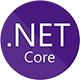•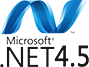•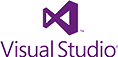•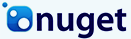•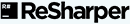•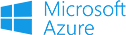## Licensing & Pricing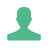### Project### Developer### Organization### Agency### SaaS## XLSX Tutorials for C# & VB .NET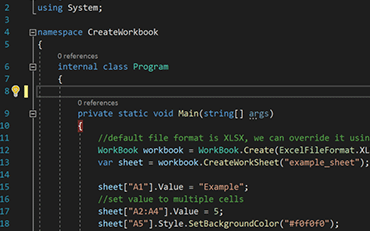C# Excel XLSX### Create XLSX Files in C#

See How Jonas uses IronXL to generate Excel Files without using Office Interop...

View Jonas' Excel File Generation Tutorial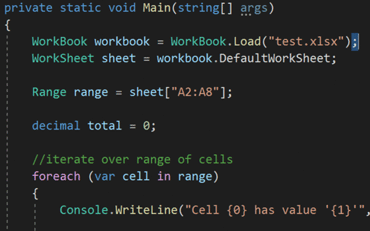C# Excel XLS### Open and Edit XLSX Files in C# or VB

Elijah shares a no-nonsense approach to reading Excel data into C# Applications...

See Elijah's Excel Reading & Editing Tutorial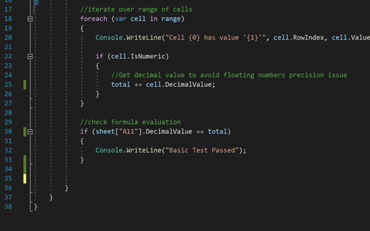C# Excel Data### How to Read XLSX Files in .NET.

Rebecca's code walkthrough for reading Excel data into a .NET application...

### Accounting and Finance Systems

• # Receipts
• # Reporting
• # Invoice Printing• # Documentation
• # Ordering & Labelling
• # Paper Replacement### Enterprise Content Management

• # Content Production
• # Document Management
• # Content Distribution### Data and Reporting Applications

• # Performance Tracking
• # Trend Mapping
• # Reports### Thousands of corporations, governments, SMEs and developers alike trust Iron software products.

Iron's team have over 10 years experience in the .NET software component market.